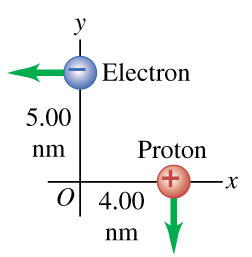# Problem: An electron and a proton are each moving at 795 km/s in perpendicular paths as shown in (Figure 1) .Part AFind the magnitude of the magnetic field the electron produces at the location of the proton.Part BFind the direction of the magnetic field the electron produces at the location of the proton.

###### FREE Expert Solution

Part A

Magnetic field:

$\overline{){\mathbf{B}}{\mathbf{=}}\frac{{\mathbf{\mu }}_{\mathbf{0}}\mathbf{qvs}\mathbf{i}\mathbf{n}\mathbf{\theta }}{\mathbf{4}\mathbf{\pi }{\mathbf{r}}^{\mathbf{2}}}}$

From Pythagoras theorem, the separation between the electron and the proton is given by:

$\begin{array}{rcl}\mathbf{r}& \mathbf{=}& \sqrt{{{\mathbf{r}}_{\mathbf{e}}}^{\mathbf{2}}\mathbf{+}{{\mathbf{r}}_{\mathbf{p}}}^{\mathbf{2}}}\\ & \mathbf{=}& \sqrt{{\mathbf{\left(}\mathbf{5}\mathbf{.}\mathbf{00}\mathbf{×}\mathbf{10}\mathbf{-}\mathbf{9}\mathbf{\right)}}^{\mathbf{2}}\mathbf{+}{\mathbf{\left(}\mathbf{4}\mathbf{.}\mathbf{00}\mathbf{×}\mathbf{10}\mathbf{-}\mathbf{9}\mathbf{\right)}}^{\mathbf{2}}}\end{array}$

98% (481 ratings)###### Problem Details

An electron and a proton are each moving at 795 km/s in perpendicular paths as shown in (Figure 1) .Part A

Find the magnitude of the magnetic field the electron produces at the location of the proton.

Part B

Find the direction of the magnetic field the electron produces at the location of the proton.